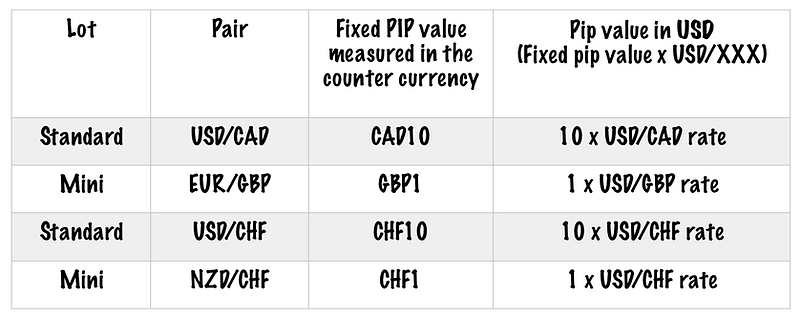# WHAT IS A "LOT" IN FOREX?

Lot

Lot is the measurement for the units of currency you buy and sell. A standard lot represents 100,000 units of any currency, whereas a mini-lot represents 10,000 and a micro-lot represents 1,000 units of any currency.

Now that you know the basics of forex, we will move on to some of the technical terms that you need to know to start your trading journey.

First, we start discussing how we measure a trade size in forex.

What is a “Lot”?

Lot is the measurement for the units of currency you buy and sell. A standard lot represents 100,000 units of any currency, whereas a mini-lot represents 10,000, and a micro-lot represents 1,000 units of any currency.Let’s assume you open a one-lot position; then your trade size equals to 100,000 units of the base currency. To calculate the value of each unit of currency that you are trading, whether it be a sell or a buy, and to calculate the total value of your one lot trade, you need to know the “Pip” value.

The change in a currency value relative to another is measured in “pips,” and the impact of the pip value on your capital is related to the amounts of lot that you trade.

For example, a one-pip movement for a standard lot corresponds with a \$10 change. Whereas one pip movement for a mini and micro lot is \$1 and \$0.1

respectively.

(More on the “pip” and its value calculation on the following topics.)